# Find the Area of Composite Shapes Easily - How to work out the Area of ANY Composite Figure.

##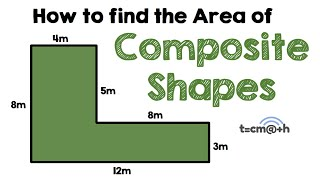By tecmath# Cylinder volume and surface area | Perimeter, area, and volume | Geometry | Khan Academy

##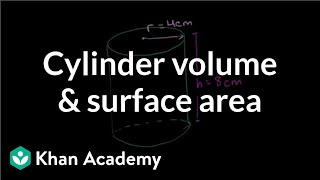By Khan Academy

The other frequently occurring shape is the cylinder. This video will introduce the cylinder and teach you the formulas for finding its surface area and volume.# Part 2 of proof of Heron's formula | Perimeter, area, and volume | Geometry | Khan Academy

##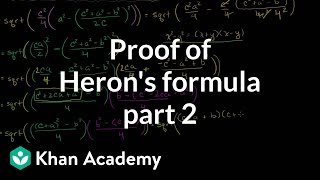By Khan Academy

Video shows that the expression in part 1 is identical to Heron's Formula. This video demonstrates how to use Heron's Formula to determine the area of a triangle while only knowing the lengths of the sides# Part 1 of proof of Heron's formula | Perimeter, area, and volume | Geometry | Khan Academy

##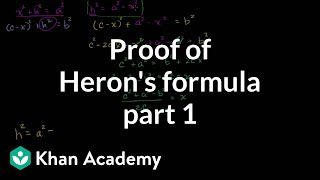By Khan Academy

In this video, the insructor explores and demonsrates the proof of Heron's Formula. The instructor demonstrates with computer software.# Heron's formula | Perimeter, area, and volume | Geometry | Khan Academy

##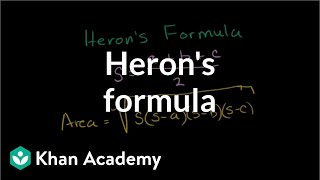By Khan Academy

Using Heron's Formula to determine the area of a triangle while only knowing the lengths of the sides. All Khan Academy content is available for free at www.khanacademy.org# Area of an equilateral triangle | Perimeter, area, and volume | Geometry | Khan Academy

##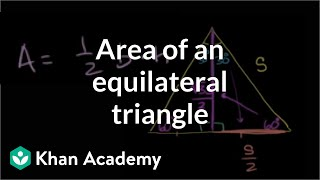By Khan Academy

Finding the formula for the area of an equilateral triangle with side s. All Khan Academy content is available for free at www.khanacademy.org# The surface area and the volume of pyramids, prisms, cylinders and cones

##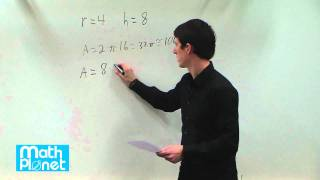By MathPlanetVideos

Find the surface area of a cylinder with the radius 4 and height 8# Surface Area and Volume of Spheres - YouTube

##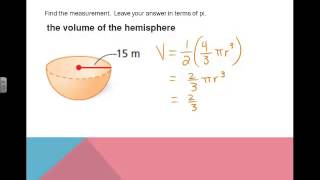By Missy McCarthy

Surface Area and Volume of Spheres - YouTube# Geometry - 19 - Pyramids - Volume, Lateral Area, and Total Area

##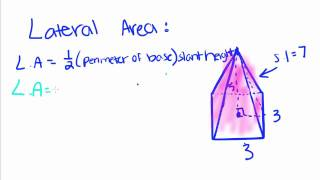By thenewboston

This video shows how to find the volume, lateral area, and total surface area of a pyramid.# Volume of a sphere | Perimeter, area, and volume | Geometry | Khan Academy

##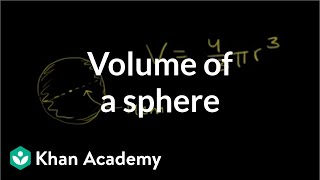By Khan Academy

"Find the volume of a sphere with a diameter of 14cm." Sal Khan solves this problem during this video clip.# Labeling parts of a circle | Perimeter, area, and volume | Geometry | Khan Academy

##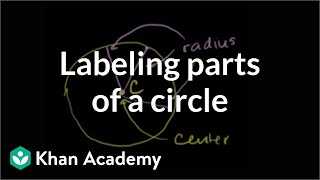By Khan Academy

Learn more: http://www.khanacademy.org/video?v=04N79tItPEA u07_l2_t3_we1 Parts of a Circle Content provided by TheNROCproject.org - (c) Monterey Institute for Technology and Education# Triangle inequality theorem | Perimeter, area, and volume | Geometry | Khan Academy

##By Khan Academy

Salman Khan offers his intuition behind the triangle inequality theorem during this six-minute video lesson.# DEPRECATED Evaluating composite functions

##By Khan Academy

Sal solves the following problem: Given that h(x)=3x and g(t)=-2t-2-h(t), find h(g(8)).# DEPRECATED Evaluating composite functions

##By Khan Academy

Sal solves the following problem: Given that h(x)=3x and g(t)=-2t-2-h(t), find h(g(8)).# DEPRECATED Evaluating composite functions

##By Khan Academy

Sal solves the following problem: Given that h(x)=3x and g(t)=-2t-2-h(t), find h(g(8)).# Evaluate composite functions from formulas

##By Khan Academy

Sal solves the following problem: Given that h(x)=3x and g(t)=-2t-2-h(t), find h(g(8)).# DEPRECATED Evaluating composite functions

##By Khan Academy

Sal solves the following problem: Given that h(x)=3x and g(t)=-2t-2-h(t), find h(g(8)).# Interesting perimeter and area problems | Perimeter, area, and volume | Geometry | Khan Academy

##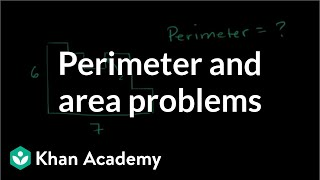By Khan Academy

problems involving perimeter and area# Perimeter and area: the basics | Perimeter, area, and volume | Geometry | Khan Academy

##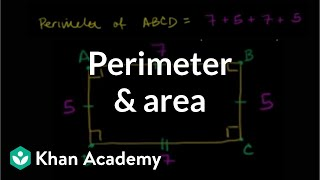By Khan Academy

perimeter and area.# Rotating 2D shapes in 3D | Perimeter, area, and volume | Geometry | Khan Academy

##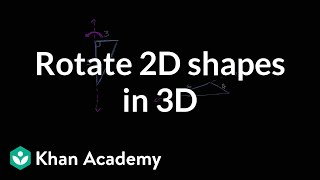By Khan Academy

This video shows the effect of rotating a 2D shape to show the relationship between 2D and 3D shapes.